## Buildings.Utilities.Psychrometrics

Library with psychrometric functions

### Information

This package contains blocks and functions for psychrometric calculations.

The nomenclature used in this package is described at Buildings.UsersGuide.Conventions.

Extends from Modelica.Icons.VariantsPackage (Icon for package containing variants).

### Package Content

Name DescriptionConstants Library of constants for psychometric functionsDensity_pTX Block to compute the density for given pressure, dry bulb temperature and moisture mass fractionPhi_pTX Block to compute the relative humidity for given pressure, dry bulb temperature and moisture mass fractionSaturationPressure Saturation pressure as a function of temperatureSaturationPressureLiquid Saturation pressure as a function of temperatureSublimationPressureIce Saturation pressure as a function of temperature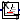TDewPoi_pW Model to compute the dew point temperature for given water vapor pressure of moist airTWetBul_TDryBulPhi Model to compute the wet bulb temperature based on relative humidityTWetBul_TDryBulXi Model to compute the wet bulb temperature based on mass fractionToDryAir Block to convert absolute humidity from [kg/kg total air] to [kg/kg dry air]ToTotalAir Block to convert absolute humidity from [kg/kg dry air] to [kg/kg total air]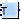X_pTphi Return steam mass fraction as a function of relative humidity phi and temperature TX_pW Humidity ratio for given water vapor pressurehSat_pTSat Calculate saturation enthalpy given a saturation (dry bulb) temperaturepW_TDewPoi Model to compute the water vapor pressure for a given dew point temperature of moist airpW_X Water vapor pressure for given humidity ratioFunctions Package with psychrometric functionsExamples Collection of models that illustrate model use and test modelsBaseClasses Package with base classes for Buildings.Utilities.Psychrometrics

##Buildings.Utilities.Psychrometrics.Density_pTX

Block to compute the density for given pressure, dry bulb temperature and moisture mass fraction### Information

Block to compute the mass density of air for given pressure, temperature and water vapor mass fraction.

Note that the water vapor mass fraction must be in kg/kg total air, and not dry air.

Extends from Modelica.Blocks.Icons.Block (Basic graphical layout of input/output block).

### Connectors

TypeNameDescription
input RealInputTDry bulb temperature [K]
input RealInputX_wWater vapor mass fraction per unit mass total air 
input RealInputpPressure [Pa]
output RealOutputdDensity [kg/m3]

### Modelica definition

block Density_pTX "Block to compute the density for given pressure, dry bulb temperature and moisture mass fraction" extends Modelica.Blocks.Icons.Block; Modelica.Blocks.Interfaces.RealInput T( final quantity="ThermodynamicTemperature", final unit="K", min=0) "Dry bulb temperature"; Modelica.Blocks.Interfaces.RealInput X_w( final unit="1", min=0) "Water vapor mass fraction per unit mass total air"; Modelica.Blocks.Interfaces.RealInput p(final quantity="Pressure", final unit="Pa", displayUnit="Pa", min = 0) "Pressure"; Modelica.Blocks.Interfaces.RealOutput d( final unit="kg/m3", final quantity="Density", final min=0) "Density"; equation d = Buildings.Utilities.Psychrometrics.Functions.density_pTX( p=p, T=T, X_w=X_w); end Density_pTX;

##Buildings.Utilities.Psychrometrics.Phi_pTX

Block to compute the relative humidity for given pressure, dry bulb temperature and moisture mass fraction### Information

Block to compute the relative humidity of air for given pressure, temperature and water vapor mass fraction.

Note that the water vapor mass fraction must be in kg/kg total air, and not dry air.

Extends from Modelica.Blocks.Icons.Block (Basic graphical layout of input/output block).

### Connectors

TypeNameDescription
input RealInputTDry bulb temperature [K]
input RealInputX_wWater vapor mass fraction per unit mass total air 
input RealInputpPressure [Pa]
output RealOutputphiRelative humidity 

### Modelica definition

block Phi_pTX "Block to compute the relative humidity for given pressure, dry bulb temperature and moisture mass fraction" extends Modelica.Blocks.Icons.Block; Modelica.Blocks.Interfaces.RealInput T( final quantity="ThermodynamicTemperature", final unit="K", min=0) "Dry bulb temperature"; Modelica.Blocks.Interfaces.RealInput X_w( final unit="1", min=0) "Water vapor mass fraction per unit mass total air"; Modelica.Blocks.Interfaces.RealInput p(final quantity="Pressure", final unit="Pa", displayUnit="Pa", min = 0) "Pressure"; Modelica.Blocks.Interfaces.RealOutput phi( final unit="1") "Relative humidity"; equation phi = Buildings.Utilities.Psychrometrics.Functions.phi_pTX( p=p, T=T, X_w=X_w); end Phi_pTX;

##Buildings.Utilities.Psychrometrics.SaturationPressure

Saturation pressure as a function of temperature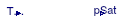### Information

Saturation pressure of water, computed from temperature, according to Wagner et al. (1993). The range of validity is between 190 and 373.16 Kelvin.

#### References

Wagner W., A. Saul, A. Pruss. International equations for the pressure along the melting and along the sublimation curve of ordinary water substance, equation 3.5. 1993. http://aip.scitation.org/doi/pdf/10.1063/1.555947?class=pdf.

Extends from Modelica.Blocks.Icons.Block (Basic graphical layout of input/output block).

### Connectors

TypeNameDescription
input RealInputTSatSaturation temperature [K]
output RealOutputpSatSaturation pressure [Pa]

### Modelica definition

block SaturationPressure "Saturation pressure as a function of temperature" extends Modelica.Blocks.Icons.Block; Modelica.Blocks.Interfaces.RealInput TSat(unit="K", displayUnit="degC", nominal=300) "Saturation temperature"; Modelica.Blocks.Interfaces.RealOutput pSat(unit="Pa", displayUnit="Pa", nominal=1000) "Saturation pressure"; equation pSat = Buildings.Utilities.Psychrometrics.Functions.saturationPressure(TSat); end SaturationPressure;

##Buildings.Utilities.Psychrometrics.SaturationPressureLiquid

Saturation pressure as a function of temperature### Information

Saturation pressure of water above the triple point temperature computed from temperature according to Wagner et al. (1993). The range of validity is between 273.16 and 373.16 Kelvin.

#### References

Wagner W., A. Saul, A. Pruss. International equations for the pressure along the melting and along the sublimation curve of ordinary water substance, equation 3.5. 1993. http://aip.scitation.org/doi/pdf/10.1063/1.555947?class=pdf.

Extends from Modelica.Blocks.Icons.Block (Basic graphical layout of input/output block).

### Connectors

TypeNameDescription
input RealInputTSatSaturation temperature [K]
output RealOutputpSatSaturation pressure [Pa]

### Modelica definition

block SaturationPressureLiquid "Saturation pressure as a function of temperature" extends Modelica.Blocks.Icons.Block; Modelica.Blocks.Interfaces.RealInput TSat(unit="K", displayUnit="degC", nominal=300) "Saturation temperature"; Modelica.Blocks.Interfaces.RealOutput pSat(unit="Pa", displayUnit="Pa", nominal=1000) "Saturation pressure"; equation pSat = Buildings.Utilities.Psychrometrics.Functions.saturationPressureLiquid(TSat); end SaturationPressureLiquid;

##Buildings.Utilities.Psychrometrics.SublimationPressureIce

Saturation pressure as a function of temperature### Information

Sublimation pressure of water below the triple point temperature, computed from temperature, according to Wagner et al. (1993). The range of validity is between 190 and 273.16 Kelvin.

#### References

Wagner W., A. Saul, A. Pruss. International equations for the pressure along the melting and along the sublimation curve of ordinary water substance, equation 3.5. 1993. http://aip.scitation.org/doi/pdf/10.1063/1.555947?class=pdf.

Extends from Modelica.Blocks.Icons.Block (Basic graphical layout of input/output block).

### Connectors

TypeNameDescription
input RealInputTSatSaturation temperature [K]
output RealOutputpSatSaturation pressure [Pa]

### Modelica definition

block SublimationPressureIce "Saturation pressure as a function of temperature" extends Modelica.Blocks.Icons.Block; Modelica.Blocks.Interfaces.RealInput TSat(unit="K", displayUnit="degC", nominal=300) "Saturation temperature"; Modelica.Blocks.Interfaces.RealOutput pSat(unit="Pa", displayUnit="Pa", nominal=1000) "Saturation pressure"; equation pSat = Buildings.Utilities.Psychrometrics.Functions.sublimationPressureIce(TSat); end SublimationPressureIce;

##Buildings.Utilities.Psychrometrics.TDewPoi_pW

Model to compute the dew point temperature for given water vapor pressure of moist air### Information

Dew point temperature calculation for moist air above freezing temperature.

The correlation used in this model is valid for dew point temperatures between 0°C and 200°C. It is the correlation from 2005 ASHRAE Handbook, p. 6.2. In an earlier version of this model, the equation from Peppers has been used, but this equation yielded about 15 Kelvin lower dew point temperatures.

Extends from Modelica.Blocks.Icons.Block (Basic graphical layout of input/output block).

### Connectors

TypeNameDescription
input RealInputp_wWater vapor partial pressure [Pa]
output RealOutputTDew point temperature [K]

### Modelica definition

block TDewPoi_pW "Model to compute the dew point temperature for given water vapor pressure of moist air" extends Modelica.Blocks.Icons.Block; Modelica.Blocks.Interfaces.RealInput p_w(final quantity="Pressure", final unit="Pa", displayUnit="Pa") "Water vapor partial pressure"; Modelica.Blocks.Interfaces.RealOutput T(start=278.15, final quantity="ThermodynamicTemperature", final unit="K", min = 0, displayUnit="degC") "Dew point temperature"; equation p_w = Buildings.Utilities.Psychrometrics.Functions.pW_TDewPoi(T=T); end TDewPoi_pW;

##Buildings.Utilities.Psychrometrics.TWetBul_TDryBulPhi

Model to compute the wet bulb temperature based on relative humidity### Information

This block computes the wet bulb temperature for a given dry bulb temperature, relative air humidity and atmospheric pressure.

If the constant `approximateWetBulb` is `true`, then the block uses the approximation of Stull (2011) to compute the wet bulb temperature without requiring a nonlinear equation. Otherwise, the model will introduce one nonlinear equation. The approximation by Stull is valid for a relative humidity of 5% to 99%, a temperature range from -20°C to 50°C and standard sea level pressure. For this range of data, the approximation error is -1 Kelvin to +0.65 Kelvin, with a mean error of less than 0.3 Kelvin.

Otherwise a calculation based on an energy balance is used. See #474 for a discussion. The model is validated in Buildings.Utilities.Psychrometrics.Examples.TWetBul_TDryBulPhi.

For a model that takes the mass fraction instead of the relative humidity as an input, see Buildings.Utilities.Psychrometrics.TWetBul_TDryBulXi.

#### References

Stull, Roland. Wet-Bulb Temperature from Relative Humidity and Air Temperature Roland Stull. Journal of Applied Meteorology and Climatology. Volume 50, Issue 11, pp. 2267-2269. November 2011 DOI: 10.1175/JAMC-D-11-0143.1

Extends from Modelica.Blocks.Icons.Block (Basic graphical layout of input/output block).

### Parameters

TypeNameDefaultDescription
replaceable package MediumModelica.Media.Interfaces.Pa...Medium model
BooleanapproximateWetBulbfalseSet to true to approximate wet bulb temperature

### Connectors

TypeNameDescription
replaceable package MediumMedium model
input RealInputTDryBulDry bulb temperature [K]
input RealInputphiRelative air humidity
input RealInputpPressure [Pa]
output RealOutputTWetBulWet bulb temperature [K]

### Modelica definition

block TWetBul_TDryBulPhi "Model to compute the wet bulb temperature based on relative humidity" extends Modelica.Blocks.Icons.Block; replaceable package Medium = Modelica.Media.Interfaces.PartialCondensingGases "Medium model"; parameter Boolean approximateWetBulb=false "Set to true to approximate wet bulb temperature"; Modelica.Blocks.Interfaces.RealInput TDryBul( start=Medium.T_default, final quantity="ThermodynamicTemperature", final unit="K", min=0) "Dry bulb temperature"; Modelica.Blocks.Interfaces.RealInput phi(min=0, max=1) "Relative air humidity"; Modelica.Blocks.Interfaces.RealInput p(final quantity="Pressure", final unit="Pa", min = 0) "Pressure"; Modelica.Blocks.Interfaces.RealOutput TWetBul( start=Medium.T_default-2, final quantity="ThermodynamicTemperature", final unit="K", min=0) "Wet bulb temperature"; protected Modelica.SIunits.Conversions.NonSIunits.Temperature_degC TDryBul_degC "Dry bulb temperature in degree Celsius"; Real rh_per(min=0) "Relative humidity in percentage"; Modelica.SIunits.MassFraction XiDryBul "Water vapor mass fraction at dry bulb state"; Modelica.SIunits.MassFraction XiSat "Water vapor mass fraction at saturation"; Modelica.SIunits.MassFraction XiSatRefIn "Water vapor mass fraction at saturation, referenced to inlet mass flow rate"; equation if approximateWetBulb then TDryBul_degC = TDryBul - 273.15; rh_per = 100*phi; TWetBul = 273.15 + TDryBul_degC * Modelica.Math.atan(0.151977 * sqrt(rh_per + 8.313659)) + Modelica.Math.atan(TDryBul_degC + rh_per) - Modelica.Math.atan(rh_per-1.676331) + 0.00391838 * rh_per^(1.5) * Modelica.Math.atan( 0.023101 * rh_per) - 4.686035; XiSat = 0; XiDryBul = 0; XiSatRefIn=0; else XiSatRefIn=(1-XiDryBul)*XiSat/(1-XiSat); XiSat = Buildings.Utilities.Psychrometrics.Functions.X_pSatpphi( pSat = Buildings.Utilities.Psychrometrics.Functions.saturationPressureLiquid(TWetBul), p = p, phi = 1); XiDryBul =Buildings.Utilities.Psychrometrics.Functions.X_pSatpphi( p = p, pSat = Buildings.Utilities.Psychrometrics.Functions.saturationPressureLiquid(TDryBul), phi = phi); (TWetBul-Buildings.Utilities.Psychrometrics.Constants.T_ref) * ( (1-XiDryBul) * Buildings.Utilities.Psychrometrics.Constants.cpAir + XiSatRefIn * Buildings.Utilities.Psychrometrics.Constants.cpSte + (XiDryBul-XiSatRefIn) * Buildings.Utilities.Psychrometrics.Constants.cpWatLiq) = (TDryBul-Buildings.Utilities.Psychrometrics.Constants.T_ref) * ( (1-XiDryBul) * Buildings.Utilities.Psychrometrics.Constants.cpAir + XiDryBul * Buildings.Utilities.Psychrometrics.Constants.cpSte) + (XiDryBul-XiSatRefIn) * Buildings.Utilities.Psychrometrics.Constants.h_fg; TDryBul_degC = 0; rh_per = 0; end if; end TWetBul_TDryBulPhi;

##Buildings.Utilities.Psychrometrics.TWetBul_TDryBulXi

Model to compute the wet bulb temperature based on mass fraction### Information

This block computes the wet bulb temperature for a given dry bulb temperature, mass fraction and atmospheric pressure.

If the constant `approximateWetBulb` is `true`, then the block uses the approximation of Stull (2011) to compute the wet bulb temperature without requiring a nonlinear equation. Otherwise, the model will introduce one nonlinear equation. The approximation by Stull is valid for a relative humidity of 5% to 99%, a temperature range from -20°C to 50°C and standard sea level pressure. For this range of data, the approximation error is -1 Kelvin to +0.65 Kelvin, with a mean error of less than 0.3 Kelvin.

Otherwise a calculation based on an energy balance is used. See #474 for a discussion.

For a model that takes the relative humidity instead of the mass fraction as an input, see Buildings.Utilities.Psychrometrics.TWetBul_TDryBulPhi.

#### References

Stull, Roland. Wet-Bulb Temperature from Relative Humidity and Air Temperature Roland Stull. Journal of Applied Meteorology and Climatology. Volume 50, Issue 11, pp. 2267-2269. November 2011 DOI: 10.1175/JAMC-D-11-0143.1

Extends from Modelica.Blocks.Icons.Block (Basic graphical layout of input/output block).

### Parameters

TypeNameDefaultDescription
replaceable package MediumModelica.Media.Interfaces.Pa...Medium model
BooleanapproximateWetBulbfalseSet to true to approximate wet bulb temperature

### Connectors

TypeNameDescription
replaceable package MediumMedium model
input RealInputTDryBulDry bulb temperature [K]
input RealInputpPressure [Pa]
output RealOutputTWetBulWet bulb temperature [K]
input RealInputXi[Medium.nXi]Species concentration at dry bulb temperature

### Modelica definition

block TWetBul_TDryBulXi "Model to compute the wet bulb temperature based on mass fraction" extends Modelica.Blocks.Icons.Block; replaceable package Medium = Modelica.Media.Interfaces.PartialCondensingGases "Medium model"; parameter Boolean approximateWetBulb=false "Set to true to approximate wet bulb temperature"; Modelica.Blocks.Interfaces.RealInput TDryBul( start=303, final quantity="ThermodynamicTemperature", final unit="K", min=0) "Dry bulb temperature"; Modelica.Blocks.Interfaces.RealInput p( final quantity="Pressure", final unit="Pa", min = 0) "Pressure"; Modelica.Blocks.Interfaces.RealOutput TWetBul( start=293, final quantity="ThermodynamicTemperature", final unit="K", min=0) "Wet bulb temperature"; Modelica.Blocks.Interfaces.RealInput Xi[Medium.nXi] "Species concentration at dry bulb temperature"; protected Modelica.SIunits.Conversions.NonSIunits.Temperature_degC TDryBul_degC "Dry bulb temperature in degree Celsius"; Real rh_per(min=0) "Relative humidity in percentage"; Modelica.SIunits.MassFraction XiSat(start=0.01, nominal=0.01) "Water vapor mass fraction at saturation"; Modelica.SIunits.MassFraction XiSatRefIn "Water vapor mass fraction at saturation, referenced to inlet mass flow rate"; parameter Integer iWat = sum({( if Modelica.Utilities.Strings.isEqual(string1=Medium.substanceNames[i], string2="Water", caseSensitive=false) then i else 0) for i in 1:Medium.nX}) "Index of water in medium composition vector"; initial equation assert(iWat > 0, "Did not find medium species 'water' in the medium model. Change medium model."); equation if approximateWetBulb then TDryBul_degC = TDryBul - 273.15; rh_per = 100 * p/ Buildings.Utilities.Psychrometrics.Functions.saturationPressure(TDryBul) *Xi[iWat]/(Xi[iWat] + Buildings.Utilities.Psychrometrics.Constants.k_mair*(1-Xi[iWat])); TWetBul = 273.15 + TDryBul_degC * Modelica.Math.atan(0.151977 * sqrt(rh_per + 8.313659)) + Modelica.Math.atan(TDryBul_degC + rh_per) - Modelica.Math.atan(rh_per-1.676331) + 0.00391838 * rh_per^(1.5) * Modelica.Math.atan( 0.023101 * rh_per) - 4.686035; XiSat = 0; XiSatRefIn=0; else XiSatRefIn=(1-Xi[iWat])*XiSat/(1-XiSat); XiSat = Buildings.Utilities.Psychrometrics.Functions.X_pSatpphi( pSat = Buildings.Utilities.Psychrometrics.Functions.saturationPressureLiquid(TWetBul), p = p, phi = 1); (TWetBul-Buildings.Utilities.Psychrometrics.Constants.T_ref) * ( (1-Xi[iWat]) * Buildings.Utilities.Psychrometrics.Constants.cpAir + XiSatRefIn * Buildings.Utilities.Psychrometrics.Constants.cpSte + (Xi[iWat]-XiSatRefIn) * Buildings.Utilities.Psychrometrics.Constants.cpWatLiq) = (TDryBul-Buildings.Utilities.Psychrometrics.Constants.T_ref) * ( (1-Xi[iWat]) * Buildings.Utilities.Psychrometrics.Constants.cpAir + Xi[iWat] * Buildings.Utilities.Psychrometrics.Constants.cpSte) + (Xi[iWat]-XiSatRefIn) * Buildings.Utilities.Psychrometrics.Constants.h_fg; TDryBul_degC = 0; rh_per = 0; end if; end TWetBul_TDryBulXi;

##Buildings.Utilities.Psychrometrics.ToDryAir

Block to convert absolute humidity from [kg/kg total air] to [kg/kg dry air]### Information

Block that converts humidity concentration from [kg/kg total air] to [kg/kg dry air].

Extends from Modelica.Blocks.Icons.Block (Basic graphical layout of input/output block).

### Connectors

TypeNameDescription
output RealOutputXiDryWater vapor concentration in [kg/kg dry air]
input RealInputXiTotalAirWater vapor concentration in [kg/kg total air]

### Modelica definition

block ToDryAir "Block to convert absolute humidity from [kg/kg total air] to [kg/kg dry air]" extends Modelica.Blocks.Icons.Block; Modelica.Blocks.Interfaces.RealOutput XiDry "Water vapor concentration in [kg/kg dry air]"; Modelica.Blocks.Interfaces.RealInput XiTotalAir "Water vapor concentration in [kg/kg total air]"; equation XiDry = XiTotalAir / (1-XiTotalAir); end ToDryAir;

##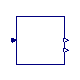Buildings.Utilities.Psychrometrics.ToTotalAir

Block to convert absolute humidity from [kg/kg dry air] to [kg/kg total air]### Information

Block that converts humidity concentration from [kg/kg dry air] to [kg/kg total air].

This block may be used, for example, to convert absolute humidity that is received from EnergyPlus to [kg/kg total air], which is the convention used by Modelica.Media.

Extends from Modelica.Blocks.Icons.Block (Basic graphical layout of input/output block).

### Connectors

TypeNameDescription
output RealOutputXiTotalAirWater vapor concentration in [kg/kg total air]
input RealInputXiDryWater vapor concentration in [kg/kg dry air]
output RealOutputXNonVaporMass fraction of remaining substances

### Modelica definition

block ToTotalAir "Block to convert absolute humidity from [kg/kg dry air] to [kg/kg total air]" extends Modelica.Blocks.Icons.Block; Modelica.Blocks.Interfaces.RealOutput XiTotalAir "Water vapor concentration in [kg/kg total air]"; Modelica.Blocks.Interfaces.RealInput XiDry "Water vapor concentration in [kg/kg dry air]"; Modelica.Blocks.Interfaces.RealOutput XNonVapor "Mass fraction of remaining substances"; equation XiTotalAir = XiDry / (1+XiDry); XNonVapor = 1 - XiTotalAir; end ToTotalAir;

##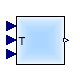Buildings.Utilities.Psychrometrics.X_pTphi

Return steam mass fraction as a function of relative humidity phi and temperature T### Information

Block to compute the water vapor concentration based on pressure, temperature and relative humidity.

If `use_p_in` is false (default option), the `p` parameter is used as atmospheric pressure, and the `p_in` input connector is disabled; if `use_p_in` is true, then the `p` parameter is ignored, and the value provided by the input connector is used instead.

Extends from Buildings.Utilities.Psychrometrics.BaseClasses.HumidityRatioVaporPressure (Humidity ratio for given water vapor pressure).

### Parameters

TypeNameDefaultDescription
Booleanuse_p_intrueGet the pressure from the input connector
Pressurep101325Fixed value of pressure [Pa]

### Connectors

TypeNameDescription
input RealInputp_inAtmospheric Pressure [Pa]
input RealInputTTemperature [K]
input RealInputphiRelative humidity (0...1)
output RealOutputX[Medium.nX]Steam mass fraction

### Modelica definition

block X_pTphi "Return steam mass fraction as a function of relative humidity phi and temperature T" extends Buildings.Utilities.Psychrometrics.BaseClasses.HumidityRatioVaporPressure; package Medium = Buildings.Media.Air "Medium model"; Modelica.Blocks.Interfaces.RealInput T(final unit="K", displayUnit="degC", min = 0) "Temperature"; Modelica.Blocks.Interfaces.RealInput phi(min = 0, max=1) "Relative humidity (0...1)"; Modelica.Blocks.Interfaces.RealOutput X[Medium.nX](each min=0, each max=1) "Steam mass fraction"; protected Modelica.SIunits.AbsolutePressure pSat "Saturation pressure"; parameter Integer i_w= sum({( if Modelica.Utilities.Strings.isEqual( string1=Medium.substanceNames[i], string2="Water", caseSensitive=false) then i else 0) for i in 1:Medium.nX}); parameter Integer i_nw = if i_w == 1 then 2 else 1 "Index for non-water substance"; parameter Boolean found = i_w > 0 "Flag, used for error checking"; initial equation assert(Medium.nX==2, "The implementation is only valid if Medium.nX=2."); assert(found, "Did not find medium species 'water' in the medium model. Change medium model."); equation pSat = Buildings.Media.Air.saturationPressure(T); X[i_w] = Buildings.Utilities.Psychrometrics.Functions.X_pSatpphi( pSat=pSat, p=p_in_internal, phi=phi); //sum(X[:]) = 1; // The formulation with a sum in an equation section leads to a nonlinear equation system X[i_nw] = 1 - X[i_w]; end X_pTphi;

##Buildings.Utilities.Psychrometrics.X_pW

Humidity ratio for given water vapor pressure### Information

Block to compute the humidity ratio for a given water vapor partial pressure.

If `use_p_in` is false (default option), the `p` parameter is used as atmospheric pressure, and the `p_in` input connector is disabled; if `use_p_in` is true, then the `p` parameter is ignored, and the value provided by the input connector is used instead.

Extends from Buildings.Utilities.Psychrometrics.BaseClasses.HumidityRatioVaporPressure (Humidity ratio for given water vapor pressure).

### Parameters

TypeNameDefaultDescription
Booleanuse_p_intrueGet the pressure from the input connector
Pressurep101325Fixed value of pressure [Pa]

### Connectors

TypeNameDescription
input RealInputp_inAtmospheric Pressure [Pa]
output RealOutputX_wSpecies concentration at dry bulb temperature
input RealInputp_wWater vapor pressure [Pa]

### Modelica definition

block X_pW "Humidity ratio for given water vapor pressure" extends Buildings.Utilities.Psychrometrics.BaseClasses.HumidityRatioVaporPressure; Modelica.Blocks.Interfaces.RealOutput X_w(min=0, max=1, nominal=0.01) "Species concentration at dry bulb temperature"; Modelica.Blocks.Interfaces.RealInput p_w(final quantity="Pressure", final unit="Pa", displayUnit="Pa", min = 0.003, start=2000, nominal=1000) "Water vapor pressure"; output Modelica.SIunits.MassFraction x_w(min=0, max=1, nominal=0.01, start=0.001) "Water mass fraction per mass of dry air"; equation X_w = Buildings.Utilities.Psychrometrics.Functions.X_pW(p_w=p_w, p=p_in_internal); x_w = X_w/(1-X_w); end X_pW;

##Buildings.Utilities.Psychrometrics.hSat_pTSat

Calculate saturation enthalpy given a saturation (dry bulb) temperature### Information

Block to compute the enthalpy of saturated air given the saturation temperature and fluid pressure.

Extends from Modelica.Blocks.Icons.Block (Basic graphical layout of input/output block).

### Connectors

TypeNameDescription
input RealInputpPressure of the fluid [Pa]
input RealInputTSatSaturation temperature of the fluid [K]
output RealOutputhSatDew point temperature of air [J/kg]

### Modelica definition

model hSat_pTSat "Calculate saturation enthalpy given a saturation (dry bulb) temperature" extends Modelica.Blocks.Icons.Block; Modelica.Blocks.Interfaces.RealInput p( final quantity="Pressure", final unit="Pa", displayUnit="bar") = 101325 "Pressure of the fluid"; Modelica.Blocks.Interfaces.RealInput TSat( final quantity="ThermodynamicTemperature", final unit="K", min = 0.0, start = 288.15, nominal = 300, displayUnit="degC") "Saturation temperature of the fluid"; Modelica.Blocks.Interfaces.RealOutput hSat( final quantity="SpecificEnergy", final unit="J/kg") "Dew point temperature of air"; protected constant Integer watIdx = 1 "Index of water"; constant Integer othIdx = 2 "Index of other species"; constant Real phiSat(min=0, max=1) = 1 "Saturation relative humidity"; Modelica.SIunits.AbsolutePressure pSat "Saturation pressure of water vapor in air at TSat"; Real XSat "Mass fractions of water and air in moist air at saturation"; equation pSat = Buildings.Utilities.Psychrometrics.Functions.saturationPressure(TSat); XSat[watIdx] = Buildings.Utilities.Psychrometrics.Functions.X_pSatpphi( pSat=pSat, p=p, phi=phiSat); XSat[othIdx] = 1 - XSat[watIdx]; hSat = Buildings.Media.Air.specificEnthalpy_pTX(p=p, T=TSat, X=XSat); end hSat_pTSat;

##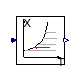Buildings.Utilities.Psychrometrics.pW_TDewPoi

Model to compute the water vapor pressure for a given dew point temperature of moist air### Information

Block to compute the water vapor pressure for a given dew point temperature.

The correlation used in this model is valid for dew point temperatures between 0°C and 30°C. It is an approximation to the correlation from 2005 ASHRAE Handbook, p. 6.2, which is valid in a wider range of temperatures and implemented in Buildings.Utilities.Psychrometrics.Functions.pW_TDewPoi. The approximation error of this simplified function is below 5% for a temperature of 0°C to 30°C. The benefit of this simpler function is that it can be inverted analytically, whereas the other function requires a numerical solution.

Extends from Modelica.Blocks.Icons.Block (Basic graphical layout of input/output block).

### Connectors

TypeNameDescription
output RealOutputp_wWater vapor partial pressure
input RealInputTDew point temperature [K]

### Modelica definition

block pW_TDewPoi "Model to compute the water vapor pressure for a given dew point temperature of moist air" extends Modelica.Blocks.Icons.Block; Modelica.Blocks.Interfaces.RealOutput p_w "Water vapor partial pressure"; Modelica.Blocks.Interfaces.RealInput T(final quantity="ThermodynamicTemperature", final unit="K", min = 0, displayUnit="degC") "Dew point temperature"; equation p_w = Buildings.Utilities.Psychrometrics.Functions.pW_TDewPoi_amb(T=T); end pW_TDewPoi;

##Buildings.Utilities.Psychrometrics.pW_X

Water vapor pressure for given humidity ratio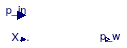### Information

Block to compute the water vapor partial pressure for a given humidity ratio.

If `use_p_in` is false (default option), the `p` parameter is used as atmospheric pressure, and the `p_in` input connector is disabled; if `use_p_in` is true, then the `p` parameter is ignored, and the value provided by the input connector is used instead.

Extends from Buildings.Utilities.Psychrometrics.BaseClasses.HumidityRatioVaporPressure (Humidity ratio for given water vapor pressure).

### Parameters

TypeNameDefaultDescription
Booleanuse_p_intrueGet the pressure from the input connector
Pressurep101325Fixed value of pressure [Pa]

### Connectors

TypeNameDescription
input RealInputp_inAtmospheric Pressure [Pa]
input RealInputX_wWater concentration at dry bulb temperature
output RealOutputp_wWater vapor pressure [Pa]

### Modelica definition

block pW_X "Water vapor pressure for given humidity ratio" extends Buildings.Utilities.Psychrometrics.BaseClasses.HumidityRatioVaporPressure; Modelica.Blocks.Interfaces.RealInput X_w(min=0, max=0.99999, nominal=0.1) "Water concentration at dry bulb temperature"; Modelica.Blocks.Interfaces.RealOutput p_w(final quantity="Pressure", final unit="Pa", displayUnit="Pa", min = 0) "Water vapor pressure"; output Modelica.SIunits.MassFraction x_w(min=0, max=1, nominal=0.1, start=0.001) "Water mass fraction per mass of dry air"; equation p_w = Buildings.Utilities.Psychrometrics.Functions.pW_X(X_w=X_w, p=p_in_internal); x_w = X_w/(1-X_w); end pW_X;